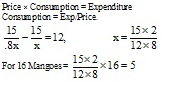# Sample Aptitude Questions of MAQ-Software

1. The difference of two numbers is 11 and one fifth of their sum is 9. The numbers are :
1. 31, 20
2. 30, 19
3. 29, 18
4. 28, 17
x − y = 11, x + y = 5 × 9 x − y = 11, x + y = 45, y = 17, x = 28
2. How many numbers between 1 and 100 are divisible by 7?
1. 9
2. 11
3. 17
4. 14
No. of divisible by 7 7, 14 --------- 98, n = a + (N - 1)d
98 = 7 + (N - 1) 7, 98 = 7 + 7N - 7
98/7= N = 14
1. What is the number which when multiplied by 13 is increased by 180?
1. 13
2. 15
3. 23
4. 35
13 × 15 = 195
2. In 24 minutes, the hour hand of a clock moves through an angle of:
1. 60°
2. 24°
3. 12°
12 hour = 360˚, 1 hr. = 360/12 = 30˚ 60 min = 30˚, 1 min 30/60 = .5˚ 24 min. = 1/2 ×24 = 12˚
3. √0.0081 is equal to :
1. 0.09
2. 0.9
3. ±0.08
4. 0.81
√0.0081 = √0.0081/10000 = √81/10000 = 9/100 =0.09
4. A reduction of 20% in the price of mangoes enables a person to purchase 12 more for Rs. 15. What was the price of 16 mangoes before reduction of price?
1. Rs. 6
2. Rs. 5
3. Rs. 7
4. Rs. 95. The average age of a man and his son is 28 years. The ratio of their ages is 3 :1 respectively. What is the man's age?
1. 30 years
2. 38 years
3. 44 years
4. 42 years
Total sum of man's age & his son's age =28 × 2 = 56 Now, the Ratio of their ages is 3 : 1.Therefore, Man's age = (3/4) × 56 = 42
So, the correct answer is option D.
6. A cyclic quadrilateral ABCD is such that AB = BC, AD = DC, AC is perpendicular to BD and ∠CAD = θ, then find the ∠ABC.
1. θ
2. θ/2
∠B + ∠D = 180° ∠A + ∠C = 180° ∠BAC = ∠BCA ∠DAC = ∠DCA ∴∠DAB = ∠DCB = 90° ∠DAC = θ
∴∠ADE = 90° - θ = ∠CDE ∴ ∠ABC = 180° – 2(90° - θ) = 2θ
7. How many integers are there between 300 and 600 that are divisible by 9?
1. 33
2. 31
3. 28
4. 25
The sequence is 306… 594
594=306+ (n-1)9⇒288= (n-1)9⇒n=33
8. 10. What will be the ratio of petrol and kerosene in the final solution formed by mixing petrol and kerosene that are present in three identical vessels in the ratio 4:1,5:2 and 6:1 respectively?
1. 166 : 22
2. 83 : 22
3. 83 : 44
4. 78 : 55
5. None of these